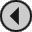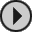## 3D CONSTRUCTION KIT

### DEFAULT KEY CONTROLS

 O = Move Forward K = Move Back I = Face Forward Z = Sidestep Left X = Sidestep Right U = U-Turn R = Move Up (Rise/Stand) F = Move Down (Fall/Crouch) W = Turn Right Q = Turn Left A = Activate Object L = Look Down P = Look Up B = Fire M = Tilt Right N = Tilt Left SPACE = Toggle Sights Mode/Movement Mode CTRL/SYMBOL SHIFT = When used in conjunction with movement keys will accelerate the movement. ESC/RUN-STOP/BREAK (CAPS SHIFT + SPACE) = Restart environment. When in the TEST screen it will take you back to the Editor.

### PALETTE VALUES

 0 = Black 9 = Green 18 = Bright Green 1 = Blue 10 = Cyan 19 = Sea Green 2 = Bright Blue 11 = Sky Blue 20 = Bright Cyan 3 = Red 12 = Yellow 21 = Lime Green 4 = Magenta 13 = White 22 = Pastel Green 5 = Mauve 14 = Pastel Blue 23 = Pastel Cyan 6 = Bright Red 15 = Orange 24 = Bright Yellow 7 = Purple 16 = Pink 25 = Pastel Yellow 8 = Bright Magenta 17 = Pastel Magenta 26 = Bright White

### Spectrum

Ink & Paper   Bright Flash
0 = Black 4 = Green 0 = No 0 = No
1 = Blue 5 = Cyan 1 = Yes 1 = Yes
2 = Red 6 = Yellow
3 = Magenta 7 = White

### Commodore 64

 0 = Black 4 = Purple 8 = Orange 12 = Grey 2 1 = White 5 = Green 9 = Brown 13 = Light Green 2 = Red 6 = Blue 10 = Light Red 14 = Light Blue 3 = Cyan 7 = Yellow 11 = Grey 1 15 = Grey 3

### SOUND EFFECTS

 0 = Silence 4 = Activate 8 = Bonus #1 12 = Door Close 1 = Ping 5 = Bump 9 = Bonus #2 2 = Buzz 6 = Fall 10 = Bonus #3 3 = Fire 7 = Fail 11 = Door Open

### RANGES OF ALLOWED VALUES

Object position X = 0-127a
Object position Y = 0-63a
Object position Z = 0-127a
Object size X = 0-127a
Object size Y = 0-63a
Object size Z = 0-127a

Viewpoint X = 0-8191b (128a x 64)
Viewpoint Y = 0-4095b (64a x 64)
Viewpoint Z = 0-8191b (128a x 64)

Numbers in Conditions = 0-255
Variables numbered 0-127 which can each store 0-255. Note that variables 112 to 127 are system variables.

a - In Object co-ordinates. 1 unit in Object co-ords = 64 units in World co-ords.

b - In World co-ords. 64 units in World co-ords = 1 unit in Object co-ords.